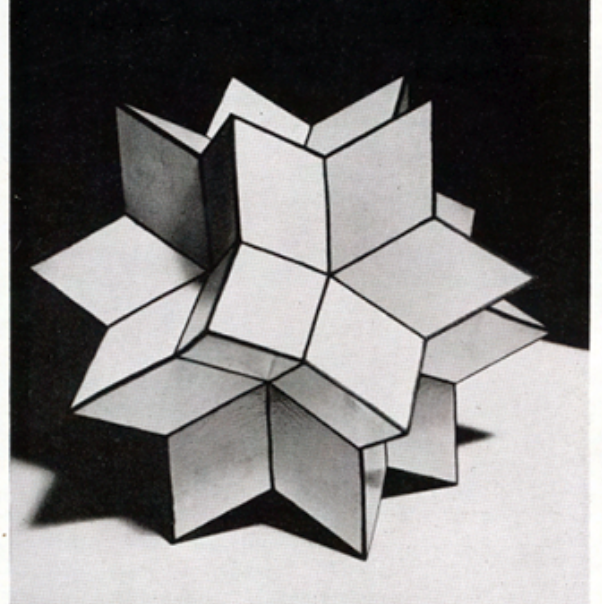# Geneticpolytopes

Algebraic geometry

## New Calabi-Yau manifolds from genetic algorithms

Physics Letters B

P. Berglund, Y. He, E. Heyes, E. Hirst, V. Jejjala, A. Lukas

Finding reflexive polytopes of dimension $n + 1$ is important as they give Calabi-Yau manifolds of dimension $n$. But only for $n = 2, 3$ and $4$ are all such polytopes classified. We use a genetic algorithm to generate these polytopes, reproducing the full set for $n = 2$ and $3$, and some for $n = 4$. We then extend to $n = 5$, and calculate normal forms to find many polytopes not currently known, revealing new Calabi-Yau $4$-folds.

Physics Letters B

P. Berglund, Y. He, E. Heyes, E. Hirst, V. Jejjala, A. Lukas